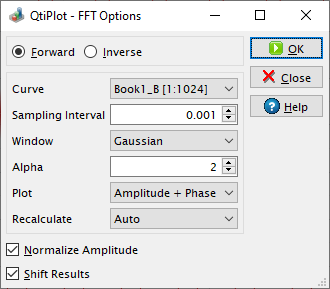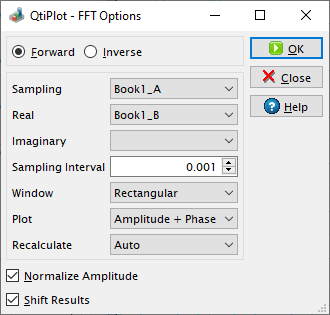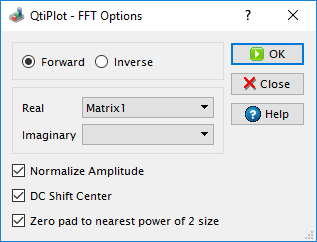# Fast Fourier Transform Dialog

The FFT... dialog box can be used on a plot, on a table or on a matrix. It is used to compute either the direct or inverse FFT. See the FFT section in the Analysis chapter for an example.

Figure 5-97. The FFT... dialog box for a curve.QtiPlot creates a hidden table with seven columns which contain the values for the frequencies, the real part (Re), the imaginary part (Im), the magnitude M = sqrt(Re2 + Im2), the amplitude (A), the phase Ph = atan(Im/Re) and the amplitude in decibels of the FFT, dB = 20log(A).

QtiPlot uses the values from the hidden table in order to create a 2D plot window with one or two layers. The type of curves displayed can be customized using the Plot list box. By default, QtiPlot creates two plot layers displaying the amplitude and the phase of the FFT.

It is possible to apply a window function to the input data, by choosing an option different then Rectangular in the Window list box. The available window functions, w(i), are listed bellow, N being the number of samples (data points) in the input signal and i the index of the data point, i = 0, 1,..., N - 1:

Rectangular

w(i) = 1. The input data is not altered.

Welch

w(i) = 1 - [2(i - 0.5*(N - 1))/(N + 1)]2

Triangular

w(i) = 2/N[N/2 - |i - N/2|]

Bartlett

w(i) = 2/(N - 1)*[(N - 1)/2 - |i - (N - 1)/2|]

Hanning

w(i) = 0.5*[1 - cos[2πi/(N - 1))]

Hamming

w(i) = 0.54 - 0.46 cos[2πi/(N - 1)]

Blackman

w(i) = 0.42 - 0.5 cos[2πi/(N - 1)] + 0.08 cos[4πi/(N - 1)]

Gaussian

w(i) = exp[-0.5(2α(i - N/2)/N)2], where α may be customized using the Alpha input box which is only available in the case of this window type. If the Alpha value is set to zero the input data is not altered.

If the Normalize Amplitude box is checked, the amplitude curve is normalized to 1. If not, the amplitude is calculated from the magnitude values using the following formulas: Ai = Mi/N for i = 0 or i = N/2 and Ai = 2Mi/N otherwise.

If the Shift Results check box is on, the frequencies are shifted in order to obtain a centered x-scale.

Figure 5-98. The FFT... dialog box for a table.When performing an FFT on a table, you must select the sampling column (X-values) and one (for simple real values) or two (for complex values) columns of Y-values. Complex numbers have the real part of the Y-values in the first column and the imaginary part of the Y-values in the second column. If Y-values are simple reals, you don't have to select a column for the imaginary part. You can leave the combo box for the second column empty in this case.

By default, the Sampling Interval corresponds to the interval between X-values. Giving a smaller value makes no sense, but you can increase this value in order to sample fewer values.

On matrices QtiPlot performs a two dimensional FFT using two sampling intervals: one that corresponds to the interval between the X-values and a second one corresponding to the interval between the Y-values. For more details about the two dimensional FFT please read the following article by Paul Bourke: http://paulbourke.net/miscellaneous/dft/.

Figure 5-99. The FFT... dialog box for a matrix.If the dimensions of the input matrix are not powers of 2, you have the option to resize it for a more efficient FFT, by checking the box Zero-pad to nearest power of 2 size. The new dimensions are calculated to be the closest powers of 2 from the initial dimensions of the matrix and the extra data values used for the FFT are set to zero.

As the result of the FFT operation on a matrix QtiPlot creates a new matrix window containing the amplitude of the FFT, along with two other matrices which contain the real and the imaginary parts of the FFT.